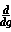# Math2.org Math Tables: Chain Rule(Math)f(g(x)) =f(g) *g(x)

Proof off(g(x)) =f(g) *g(x) from the definition

We can use the definition of the derivative:f(x) = limd-->0 f(x+d)-f(x)d
Therefore,f(g(x)) can be written as such:f(g(x)) = df/dx = (f(g(x+d) - f(g(x))/d
df/dx * 1/(dg/dx) = [ (f(g(x+d) - f(g(x))/d ] * [ d/(g(x+d) - g(x)) ]
= ( f(g(x+d))-f(g(x)) )/(g(x+d)-g(x)) = df/dg
df/dx = df/dg * dg/dx